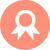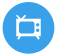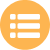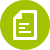## 二建-建筑工程## 监理案例分析# 最新湖南长沙升降机司机真题

A.开机检查

B.试运行检查

C.班后检查

D.班前检查

A.安全预防措施

B.安全控制措施

C.安全保护措施

D.安全防护措施

A.吊笼的外框

B.吊笼的内框

C.吊笼的界限

D.吊笼的尺寸

A.不允许

B.禁止

C.严禁

D.不得

A.吊笼冲顶

B.吊笼越位

C.吊笼越程

D.吊笼失控

A.载荷分布均匀

B.载荷集中均匀

C.载荷四周均匀

D.载荷堆放均匀

A.关闭电源箱

B.关闭电源

C.切断电源

D.关闭开关箱

A.4ω

B.6ω

C.8ω

D.10ω

A.齿条螺栓松动

B.对接阶差过大

C.导轨架未变形

D.齿轮严重磨损

A.了解

B.掌握

C.全部

D.熟悉

A.电液连锁

B.液压控制

C.机电连控

D.机电联锁

A.允许

B.禁止

C.严禁

D.不得

A.制动轮径向跳动严重超差

B.制动弹簧过松

C.电磁松闸器缺陷

D.电磁松闸器松闸不到位

A.更换失效零件并紧固锁紧螺母

B.调紧制动器弹簧

C.清理制动器油垢

D.调整制动衬料与制动轮之间的间隙

A.对称

B.对重

C.重块

D.平衡重

A.紧固电机固定装置

B.更换电机橡胶垫

C.取消电机橡胶垫

D.紧固减速箱与传动板连接螺栓

A.标准节

B.导架节

C.导梁节

D.导轨节

A.安全装置

B.制动装置

C.电气系统

D.金属结构

A.建筑施工材料

B.有毒有害物品

C.易燃易爆物品

D.障碍物

A.齿条齿条式施工升降机

B.液压式施工升降机

C.电动式施工升降机

D.齿轮式施工升降机

A.9.8级

B.5.8级

C.7.8级

D.8.8级

A.30mm

B.40mm

C.50mm

D.60mm

A.设计型式

B.使用功能

C.结构型式

D.传动型式

A.实际载重量

B.额定载重量

C.核定载重量

D.设计载重量

A.装入吊笼

B.装入吊篮

C.装入货厢

D.装入容器

A.封闭实施

B.封闭屏障

C.安全平网

D.防护棚

A.架体通道

B.上人通道

C.升降通道

D.工作通道

A.控制措施

B.保护措施

C.防护措施

D.安全措施

A.建筑施工材料

B.有毒有害物品

C.易燃易爆物品

D.化学有毒物品

A.建设单位

B.安装单位

C.使用说明书

D.专项施工方案

A.≤90db

B.≤80db

C.≤85db

D.≤86db

A.90m

B.120m

C.110m

D.100m

A.门把

B.门栓

C.门控

D.门锁

A.防护围栏

B.防护层门

C.防护装置

D.防护层站

A.20%

B.22%

C.25%

D.18%

A.防雷接地保护

B.重复保护

C.防雷接零保护

D.相互接地保护

A.安全警示标志

B.指示标志

C.警告标志

D.安全提示标志

A.限制开关

B.行程开关

C.限位开关

D.极限开关

A.应立即切断电源

B.应立即停机

C.应立即报修

D.应立即检查

A.教育内容

B.培训内容

C.考核内容

D.招聘内容

A.现场施工人员

B.施工作业人员

C.特种作业人员

D.安全管理人员

A.0.6t

B.0.7t

C.0.5t

D.0.8t

A.便于操作

B.安全操作

C.利于操作

D.适应操作

A.匀速式安全器

B.防坠安全器

C.瞬时式安全器

D.渐进式安全器

A.15%

B.20%

C.15%

D.10%

A.变型更新

B.设计更新

C.设计变形

D.更新换代

A.运行的行为

B.操作的行为

C.安全的行为

D.工作的行为

A.ssb320b

B.ssb320a

C.ssb320c

D.ssb300a

A.活载荷

B.部分载荷

C.全部载荷

D.所有载荷

A.安全锁

B.安全钳

C.安全钩

D.安全器

A.按规定调整偏心轴,使滚轮与立柱管间隙为0.8mm(0.5mm)

B.驱动齿轮磨损超标应当更换驱动齿轮

C.减速器轴弯曲应当更换减速器轴

D.齿条损坏或齿条磨损过渡

E.调整滚轮保证齿线平行,齿侧隙保持0.2-0.5mm

A.警报系统是正常

B.地面防护围栏内有无杂物

C.吊笼顶部有无杂物

D.变速箱有无异常发热及噪声

E.地面通道防护棚

A.制动器制动力矩不足

B.传动板未设置齿轮防脱轨挡块

C.防坠安全器安装不牢固

D.背轮结构缺陷

E.传动板结构稳固

A.钢丝绳

B.手拉葫芦

C.千斤顶

D.缆风绳

E.起重机

A.地面防护围栏不低于1.8m

B.地面防护围栏不低于1.6m

C.当吊笼位于底部时围栏门方能开启

D.围栏门开启后吊笼不能启动

E.围栏门开启后吊笼可以启动

A.切断控制电路

B.层站稳定

C.制动器制动

D.打开控制电路

E.吊笼停止

A.色泽

B.磨损

C.锈蚀

D.裂纹

E.变形

A.出租单位

B.安装单位

C.监理单位

D.使用单位

E.分包单位

A.索具

B.工具

C.辅助用具

D.各种零配件

E.电缆

A.液压泵

B.电动机

C.联轴器

D.减速器

E.齿轮

A.无明显塑性变形

B.无裂纹和严重锈蚀

C.焊接部位无缺陷

D.外表颜色新颖

E.弯曲无影响

A.脱皮

B.裸露

C.五芯

D.接头

E.铝芯

A.对重导轨

B.对重体

C.天轮

D.钢丝绳

E.齿轮

A.补充齿轮油

B.必要时更换输出轴

C.更换齿轮油

D.检查或更换蜗轮.蜗杆

E.检查.修复联轴节.更换轴承

A.组

B.型

C.制造商

D.特性

E.主参数

A.统一指挥

B.明确分工

C.各自为阵

D.各负其责

E.起重服从吊车司机

A.大雨

B.大雪

C.大雾

D.5级风力

E.4级风力

A.清扫基础表面

B.清除锈蚀

C.清除预留孔中积水

D.清除杂物

E.清除润滑脂

A.传动齿轮

B.防坠安全器齿轮

C.防坠安全器齿条

D.防坠安全器固定

E.安全电压

A.构件刚度

B.构件强度

C.稳定性

D.平衡性

E.承载性

A.正确

B.错误

A.正确

B.错误

A.正确

B.错误

A.正确

B.错误

A.正确

B.错误

A.正确

B.错误

A.正确

B.错误

A.正确

B.错误

A.正确

B.错误

A.正确

B.错误

A.正确

B.错误

A.正确

B.错误

A.正确

B.错误

A.正确

B.错误

A.正确

B.错误

A.正确

B.错误

A.正确

B.错误

A.正确

B.错误

A.正确

B.错误

A.正确

B.错误

2023版湖北省施工升降机司机预习题

2023版施工升降机司机考核考题
##### 版权声明

 留言与评论（共有 0 条评论）

ock123.com

### 蜀ICP备18034711号.